# Triple_Axis_Accelerometer_FXLN83XX_Series-DFRobot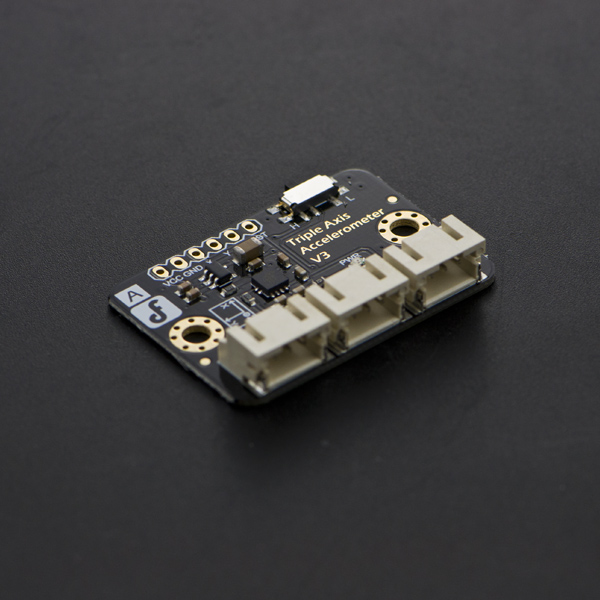## Introduction

3-Axis acceleration sensor is an electronic equipment which could measure the acceleration during the object motion. It could help you to analyse the target motion position or direction. The typical interactive application could be the posture recognition and action recognition, such as WII game applications. These video game device are all using internal acceleration sensor, calculate the current motion direction by conversion algorithm. And most of the recent smart device are based the same theory, like Misfit, Microsoft band .etc.

FXLN83XXQR1 family is a low power consumption with high precision sensor. The highest bandwidth could arrive 2.7kHz. And analog output could be compatible with most MCU.

## Specification

• Power Supply: 3.3-8V;
• Optional Sensitivity: ±2g/8g (FXLN8361QR1 & FXLN8371QR1); ±4g/16g (FXLN8362QR1 & FXLN8372QR1);
• Low power consumption (180 μA working current);
• High sensitivity;
• Low-pass filter with internal signal processing functions;
• Stable performance, shock-proof capability.
• Dimensions: 37.44X26.43mm

## User Manual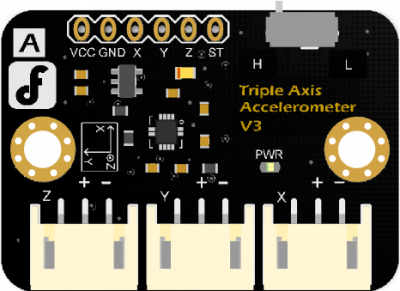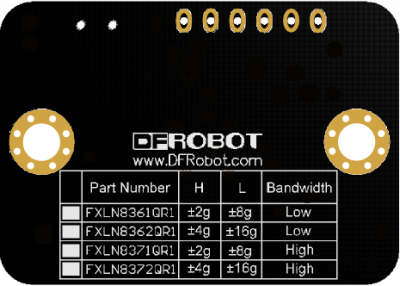SKU Chip H L Bandwidth
SEN0178 FXLN8361 ±2g ±8g Low
SEN0179 FXLN8362 ±4g ±16g Low
SEN0180 FXLN8371 ±2g ±8g High
SEN0181 FXLN8372 ±4g ±16g High
Note: "H" = High resolution, Low range; "L" = Low resolution, High range

There is a sensor table on the back of the PCB, it will show your sensor chip type, measurement range and bandwidth. You could select the measurement range via the range switch.

`E.g. If your sensor chip type is “FXLN8361QR1”; When the switch is "H", the measure range will be "±2g"; when the switch is "L", the range will be "±8g".`

## Tutorial

In this section, we will teach you how to read the correct accelerated velocity. (Sample: SEN0178)

### Connection Diagram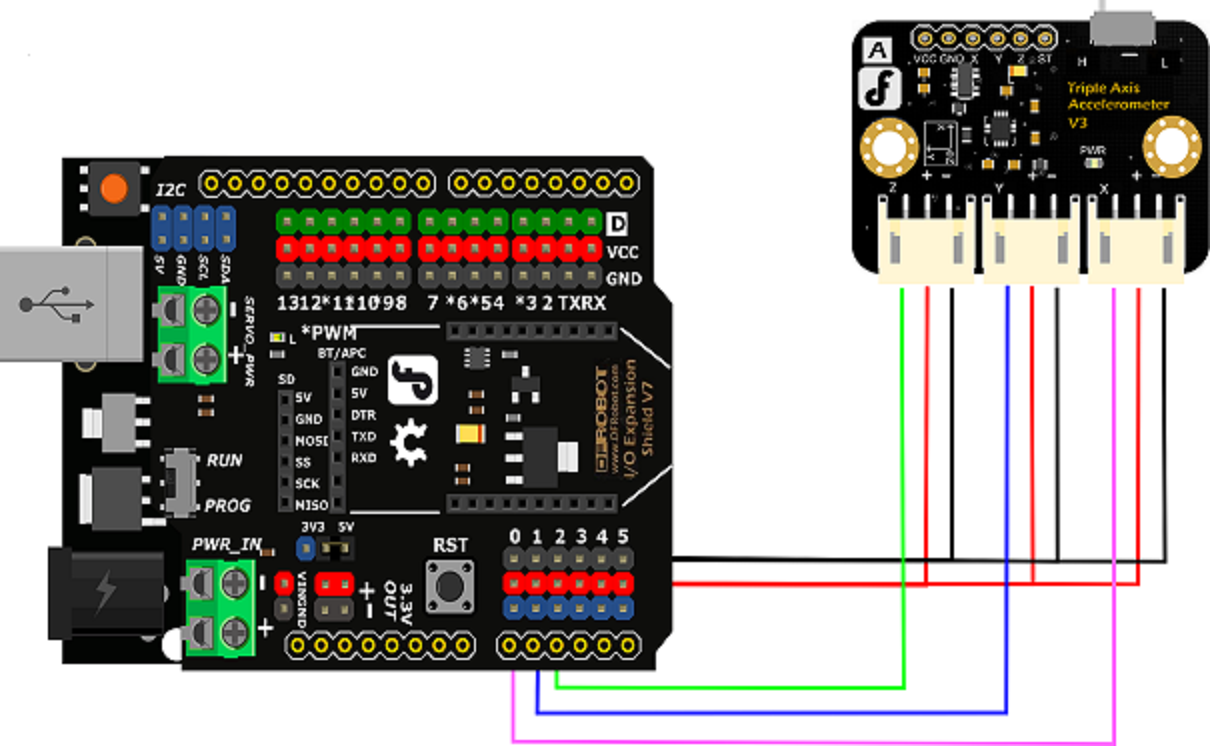### Data Calibration

Due to sensor individual difference, we need make a calibration for each module. (Or you could skip this step, and test the sensor directly.)

• Step 1

Turn the switch to "H" position, and connect the sensor according to the connection diagram. Upload the testing code to Arduino.

``````/*
DFRobot 3-axis calibration code

X——A0
Y——A1
Z——A2

*/

void setup()
{
Serial.begin(9600);
}
void loop()
{
int x,y,z;
Serial.print("x= ");
Serial.print(x*5/1024.0,3);
Serial.print(',');
Serial.print("y= ");
Serial.print(y*5/1024.0,3);
Serial.print(',');
Serial.print("z= ");
Serial.println(z*5/1024.0,3);
delay(500);
}``````
• Step 2

Leave sensor resting horizontally on the desktop, keep the positive direction of Z-axis upwards. Open the IDE serial monitor, and record a set of data. E.g.:

`x= 0.776,y= 0.776,z= 1.157`

Similarly, leave sensor resting horizontally on the desktop, keep the negative direction of Z-axis upwards. Open the IDE serial monitor, and record another set of data. E.g.:

`x= 0.776,y= 0.781,z= 0.688`

Now, you could observe two sets of data, and you will find the value on X-axis & Y-axis are equal or differ very little, but the value on Z-axis are quite different. This is because there is no accelerated velocity on X-axis and Y-axis, when the sensor is resting horizontally. The module is only forced by gravity, and its direction is always downward. With different posture, the gravitational acceleration stays the same. In physics, we call it "g". So, when the Z-axis upward, the value z should be "z=Z+g"; when the Z-axis downward, it will be "z=Z+g". You could calculate the initial value of "Z", and the corresponding value of "g".

`Z=(1.157+0.688)/2=0.923 mV` `g=(1.157-0.688)/2=0.235 mV/g`

Similarly, you could get sensor initial value "X" & "Y" and corresponding value of "g" on X-axis and Y-axis.

Note: The gravitational acceleration are always downward, and its value are only related to the local gravity. We regulate its direction as acceleration positive direction. For example: when the object is moving upward with the acceleration of "a", its acceleration will be "g+a".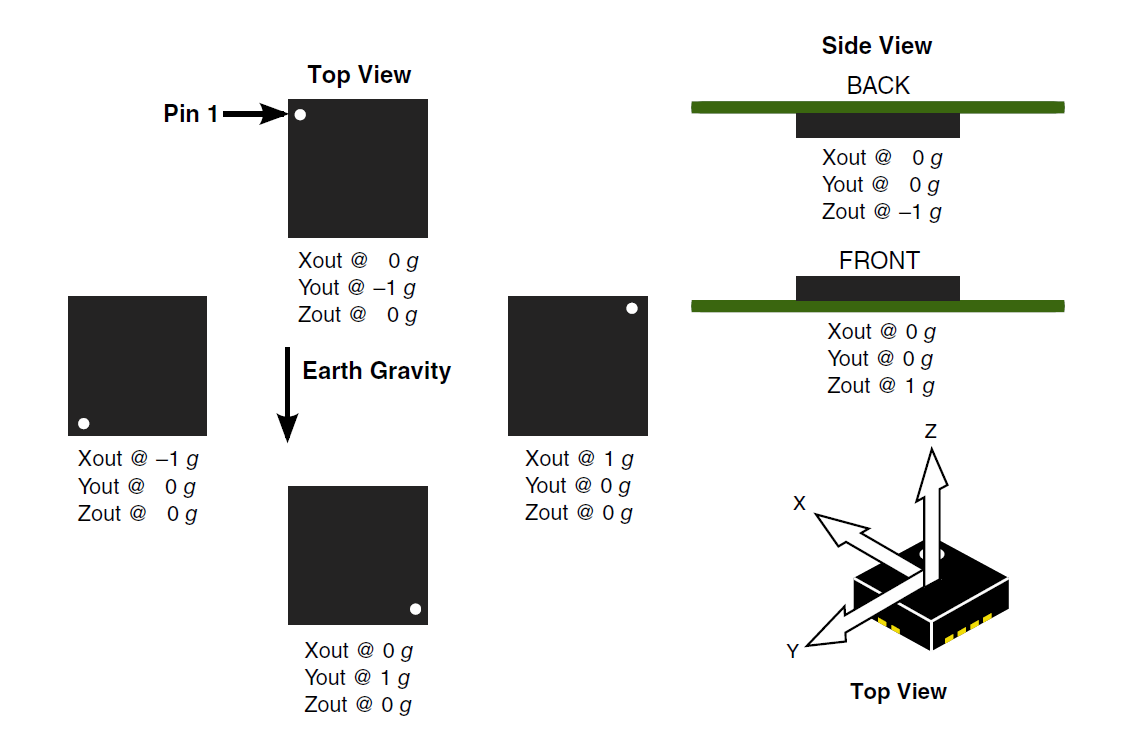• Step 3

After the calibration, we have gotten every axis initial value and corresponding value of "g". Upload the sample code, we will get the perfect acceleration velocity.

Note: In order to get the accurate data, we need do the calibration on each axis.

### Sample Code

For Arduino

``````float X=0.774;  //replace your value

void setup()
{
Serial.begin(9600);    //Baudrate 9600
}
void loop()
{
int x,y,z;
Serial.print("x= ");
Serial.print((x*5/1024.0 - X)/gx,2);
Serial.print(',');
Serial.print("y= ");
Serial.print(((y*5/1024.0- Y)/gy),2);
Serial.print(',');
Serial.print("z= ");
Serial.println(((z*5/1024.0-Z)/gz),2);
delay(100);
}``````

Open Arduino IDE, copy the sample code to IDE window, click "Upload".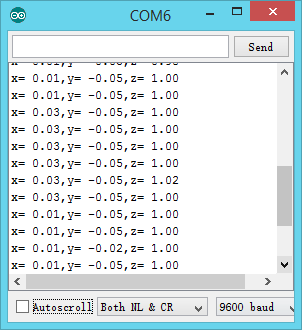Now, you can see that there is only a "g" on Z-axis, when you leave it resting horizontally.

For Micropython

``````from machine import ADC,Pin
import time

X=0.774
gx=0.237

Y=0.781
gy=0.21

Z=0.923
gz=0.235

while True:
print("x=",(x*5/1024.0 - X)/gx)
print("y=",(y*5/1024.0 - Y)/gy)
print("z=",(z*5/1024.0 - Z)/gz)
time.sleep_ms(50)``````

## FAQ

Q&A Some general Arduino Problems/FAQ/Tips
Q1 How do I make it work on the other range?
A The theory is totally same. The calibration could be applied on every range.
A Any question and more cool ideas to share, please visit DFRobot Forum

## More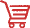Get Triple Axis Accelerometer - FXLN8361 from DFRobot Store or DFRobot Distributor.